Main Content

# Using Scale Factors to Facilitate Weight Tuning

This example shows how to specify scale factors in MPC controller to make weight tuning easier.

### Define Plant Model

The discrete-time, linear, state-space plant model has 10 states, 5 inputs, and 3 outputs.

```[plant,Ts] = mpcscalefactor_model; [ny,nu] = size(plant.D);```

The plant inputs include manipulated variable (MV), measured disturbance (MD) and unmeasured disturbance (UD). The plant outputs include measured outputs (MO) and unmeasured outputs (UO).

```mvindex = [1, 3, 5]; mdindex = 4; udindex = 2; moindex = [1 3]; uoindex = 2; plant = setmpcsignals(plant,'MV',mvindex,'MD',mdindex,'UD',udindex,'MO',moindex,'UO',uoindex);```

The nominal values and operating ranges of plant model are as follows:

• Input 1: nominal value is 100, range is [50 150]

• Input 2: nominal value is 10, range is [5 15]

• Input 3: nominal value is 0.01, range is [0.005 0.015]

• Input 4: nominal value is 0.1, range is [0.05 0.15]

• Input 5: nominal value is 1, range is [0.5 1.5]

• Output 1: nominal value is 0.01, range is [0.005 0.015]

• Output 2: nominal value is 1, range is [0.5 1.5]

• Output 3: nominal value is 100, range is [50 150]

Use `lsim` command to run an open loop linear simulation to verify that plant outputs are within the range and their average are close to the nominal values when input signals vary randomly around their nominal values.

```Unominal = [100;10;0.01;0.1;1]; Ynominal = [0.01;1;100]; Uspan = Unominal; Yspan = Ynominal; t = (0:1000)'*Ts; nt = length(t); Uol = (rand(nt,nu)-0.5).*(ones(nt,1)*Uspan'); % design input signal Yol = lsim(plant,Uol,t); % compute plant output fprintf('The difference between average output values and the nominal values are %.2f%%, %.2f%%, %.2f%% respectively.\n',... abs(mean(Yol(:,1)))/Ynominal(1)*100,abs(mean(Yol(:,2)))/Ynominal(2)*100,abs(mean(Yol(:,3)))/Ynominal(3)*100);```
```The difference between average output values and the nominal values are 2.25%, 3.53%, 2.47% respectively. ```

### Evaluate MPC with Default MPC Weights

When plant input and output signals have different orders of magnitude, default MPC weight settings often give poor performance.

Create an MPC controller with default weights:

• `Weight.MV = 0`

• `Weight.MVRate = 0.1`

• `Weight.OV = 1`

`C = mpc(plant);`
```-->The "PredictionHorizon" property of "mpc" object is empty. Trying PredictionHorizon = 10. -->The "ControlHorizon" property of the "mpc" object is empty. Assuming 2. -->The "Weights.ManipulatedVariables" property of "mpc" object is empty. Assuming default 0.00000. -->The "Weights.ManipulatedVariablesRate" property of "mpc" object is empty. Assuming default 0.10000. -->The "Weights.OutputVariables" property of "mpc" object is empty. Assuming default 1.00000. ```
```Xnominal = zeros(10,1); Unominal(udindex) = 0; % Nominal values for unmeasured disturbance must be 0 C.Model.Nominal = struct('X',Xnominal,'DX',Xnominal,'Y',Ynominal,'U',Unominal);```

First, test a sequence of step setpoint changes in three reference signals.

```nStepLen = 15; T1 = nStepLen*ny; r1 = ones(T1,1)*Ynominal(:)'; ii = 1; for i = 1:ny r1(ii:end,i) = r1(ii:end,i) + Ynominal(i); ii = ii + nStepLen; end sim(C,T1,r1)```
```-->The "Model.Disturbance" property of "mpc" object is empty: Assuming unmeasured input disturbance #2 is integrated white noise. -->Assuming output disturbance added to measured output channel #1 is integrated white noise. Assuming no disturbance added to measured output channel #3. -->The "Model.Noise" property of the "mpc" object is empty. Assuming white noise on each measured output channel. ```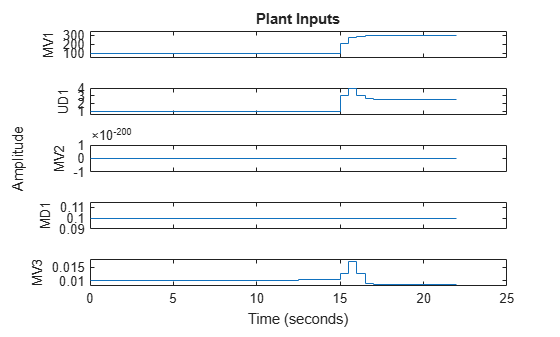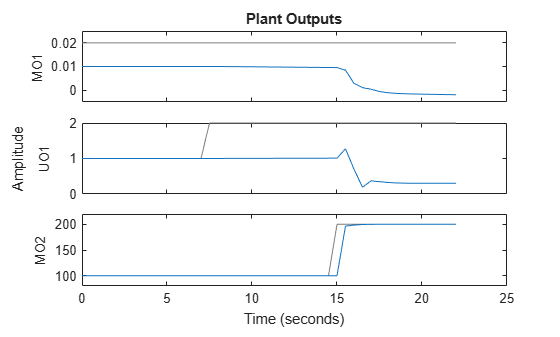The tracking response of the first output is poor. The reason is that its range is small compared to the other outputs. If the default controller tuning weights are used, the MPC controller does not pay much attention to regulating this output because the associated penalty is so small compared to the other outputs in the objective function.

Second, test the unmeasured disturbance rejection.

```SimOpt = mpcsimopt; SimOpt.UnmeasuredDisturbance = Uspan(udindex)'; T2 = 100; r2 = ones(T2,1)*Ynominal(:)'; sim(C,T2,r2,[],SimOpt)```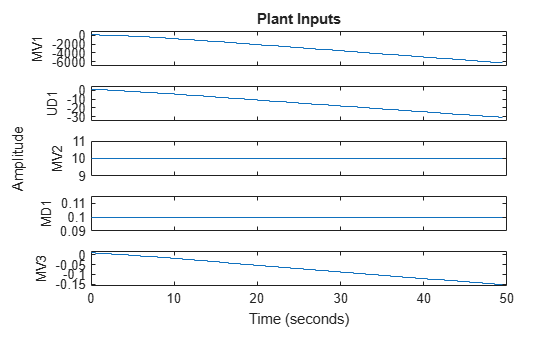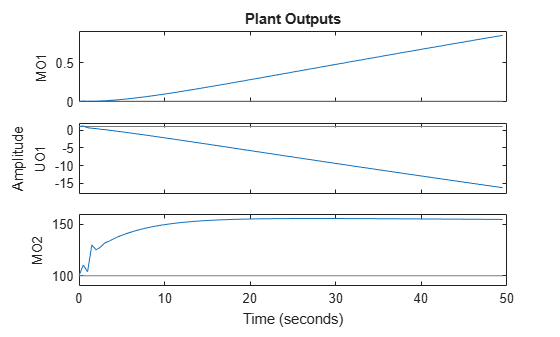The disturbance rejection response is also poor. None of the outputs return to their setpoints.

### Evaluate MPC with Default MPC Weights After Specifying Scale Factors

Specifying input and output scale factors for the MPC controller:

• Improves the numerical quality of the optimization and state estimation calculations.

• Makes it more likely that the default tuning weights will achieve good controller performance.

Copy the MPC controller with default weights.

`C2 = C;`

To specify scale factors, it is good practice to use the expected operating range of each input and output.

```for i = 1:length(mvindex) C2.MV(i).ScaleFactor = Uspan(mvindex(i)); end nmd = length(mdindex); for i = 1:nmd C2.D(i).ScaleFactor = Uspan(mdindex(i)); end for i = 1:length(udindex) C2.D(i+nmd).ScaleFactor = Uspan(udindex(i)); end for i = 1:ny C2.OV(i).ScaleFactor = Yspan(i); end```

Repeat the first test, which is a sequence of step setpoint changes in three reference signals.

`sim(C2,T1,r1)`
```-->The "Model.Disturbance" property of "mpc" object is empty: Assuming unmeasured input disturbance #2 is integrated white noise. -->Assuming output disturbance added to measured output channel #1 is integrated white noise. Assuming no disturbance added to measured output channel #3. -->The "Model.Noise" property of the "mpc" object is empty. Assuming white noise on each measured output channel. ```Repeat the second test, which is an unmeasured disturbance.

`sim(C2,T2,r2,[],SimOpt)`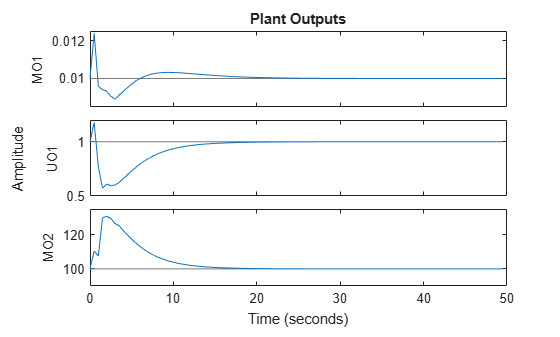Both setpoint tracking and disturbance rejection responses are good even without tuning MPC weights.

## Support

#### Implementing an Adaptive Cruise Controller with Simulink

Download technical paper